# Q4. Suppose a duopoly is characterized by the following profits: if the two firms collude and...

Q4. Suppose a duopoly is characterized by the following profits: if the two firms collude and charge the joint profit-maximizing price, they each earn a profit equal to 1500 in each period; if the two firms charge the Cournot–Nash price, they each earn a profit equal to 1200 in each period; and if one firm defects while the other charges the joint profit-maximizing price, the firm that defects earns 3000 and the other earns 0. [20 marks] a) [3 marks] What are each firm’s strategies and the payoff matrix of this game? b) [7 marks] What is the Nash equilibrium? Is this game a prisoner’s dilemma? Explain why or why not. c) [10 marks] Now suppose both firms adopt the following the strategies: (1) Start by cooperating with each firm charging the joint profit-maximizing collusive price; (2) continue to sell at the joint profit-maximizing outputs and price unless the other firm increases its output and lowers its price, in which case produce the Cournot–Nash quantity and charge the Cournot–Nash price forever. What discount rates will sustain collusion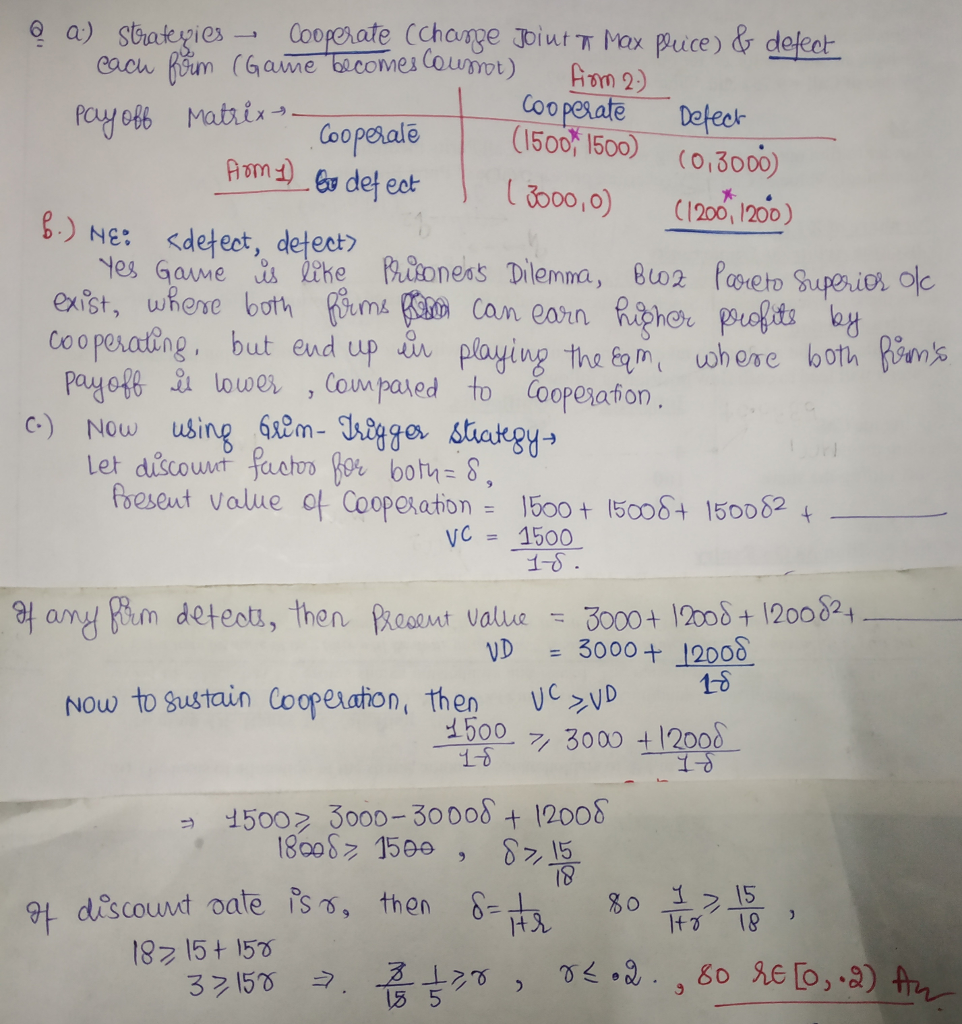#### Earn Coin

Coins can be redeemed for fabulous gifts.

Similar Homework Help Questions
• ### Suppose the two firms cannot collude and instead compete in the Cournot Model in the market...

Suppose the two firms cannot collude and instead compete in the Cournot Model in the market described in question 1 (market demand is still Q=18-P) with the same cost (C(Q)=1/2 *Q^2). Set up firm 1’s profit maximization. Solve for firm 1’s best response function. Solve for firm 1’s quantity, firm 2’s quantity, the equilibrium market quantity, and price. Show your work. Is this a Nash equilibrium? Do consumers prefer the Cournot competition equilibrium over the collusion of the two firms...

• ### 3. Suppose the two firms cannot collude and instead compete in the Cournot Model in the...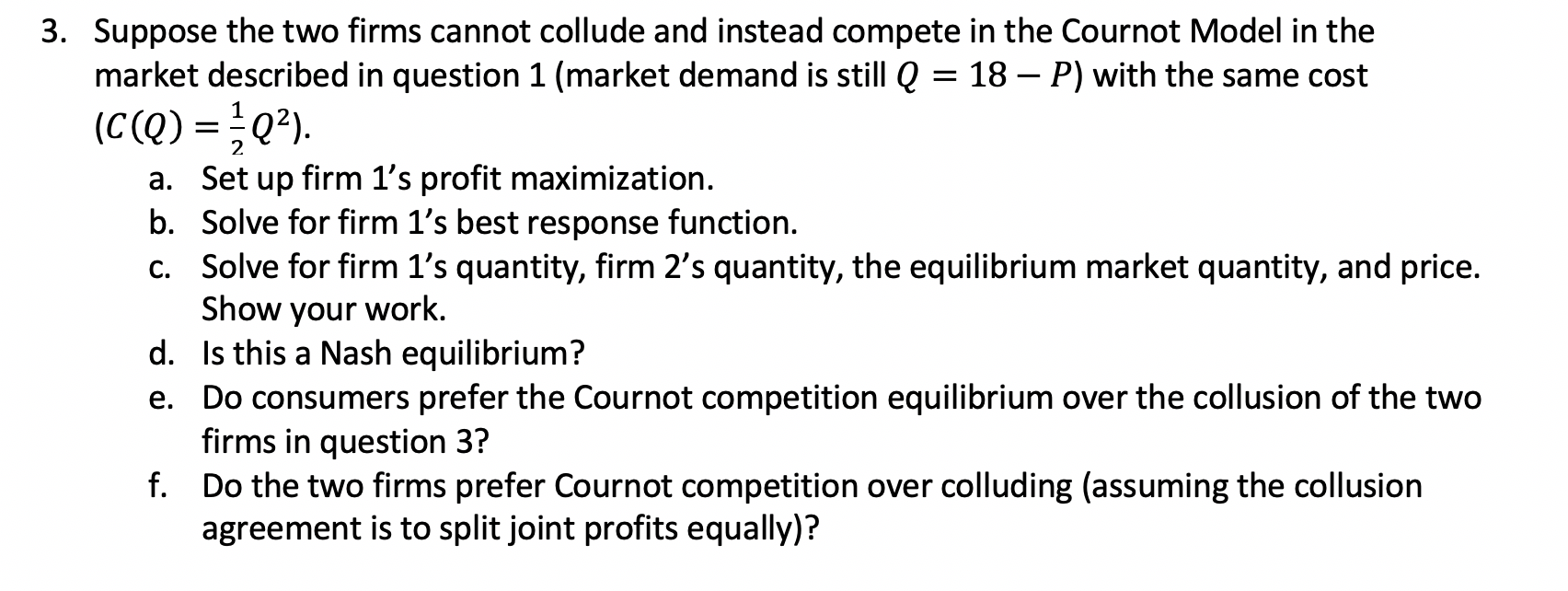3. Suppose the two firms cannot collude and instead compete in the Cournot Model in the market described in question 1 (market demand is still Q = 18 – P) with the same cost (C(Q)=Q2). a. Set up firm 1's profit maximization. b. Solve for firm 1's best response function. C. Solve for firm 1's quantity, firm 2's quantity, the equilibrium market quantity, and price. Show your work. d. Is this a Nash equilibrium? e. Do consumers prefer the Cournot...

• ### Question 5 Demand in a market dominated by two firms (a Cournot duopoly) is determined according...

Question 5 Demand in a market dominated by two firms (a Cournot duopoly) is determined according to: P = 200 – 2(Q1 + Q2), where P is the market price, Q1 is the quantity demanded by Firm 1, and Q2 is the quantity demanded by Firm 2. The marginal cost and average cost for each firm is constant; AC=MC = \$60. The cournot-duopoly equilibrium profit for each firm is _____. Hint: Write your answer to two decimal places. QUESTION 6...

• ### Consider the following payoff matrix for a game in which two firms attempt to collude under...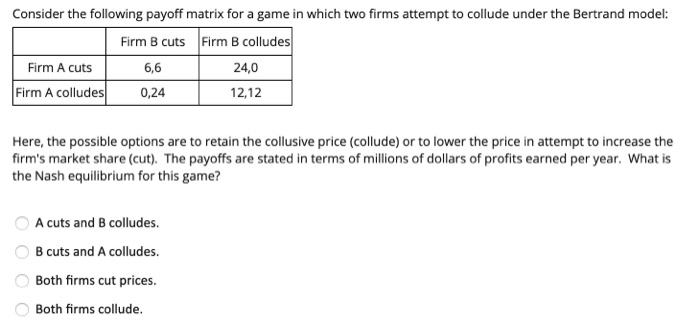Consider the following payoff matrix for a game in which two firms attempt to collude under the Bertrand model: Firm B cuts Firm B colludes Firm A cuts 6,6 24,0 Firm A colludes 0,24 L 12,12 Here, the possible options are to retain the collusive price (collude) or to lower the price in attempt to increase the firm's market share (cut). The payoffs are stated in terms of millions of dollars of profits earned per year. What is the Nash...

• ### Cournot Equillibrium Why is the Cournot equilibrium an equilibrium? O A. Given the other firm's level...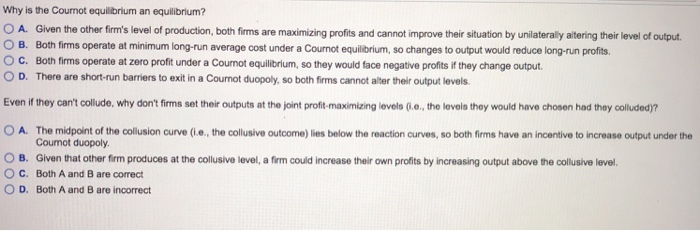Cournot Equillibrium Why is the Cournot equilibrium an equilibrium? O A. Given the other firm's level of production, both firms are maximizing profits and cannot improve their situation by u O B. Both firms operate at minimum long-run average cost under a Cournot equilibrium, so changes to output would reduce long-run profits O c. Both firms operate at zero profit under a Cournot equilibrium, so they would face negative profits if they change output. O D. There are short-run barriers...

• ### 5. Cournot Competition Consider a Coumot duopoly model. Suppose that market demand is P-a-qi Also suppose that the cost functions of the two firms are TG (q) = q, and T( (a) Write the profit func...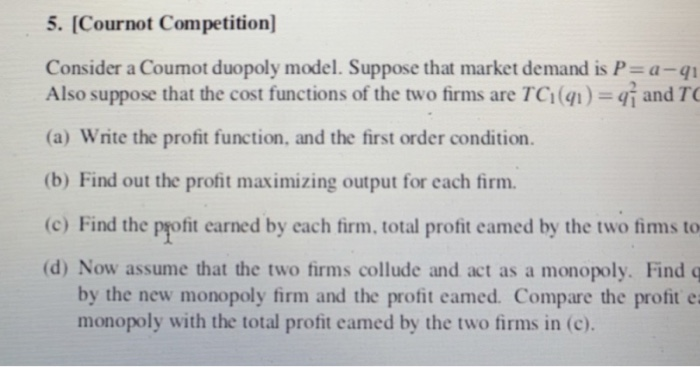5. Cournot Competition Consider a Coumot duopoly model. Suppose that market demand is P-a-qi Also suppose that the cost functions of the two firms are TG (q) = q, and T( (a) Write the profit function, and the first order condition. (b) Find out the profit maximizing output for each firm. (c) Find the pofit earned by each firm, total profit eamed by the two fims to (d) Now assume that the two firms collude and act as a monopoly....

• ### 1. Let's make a deal. Now suppose the two firms could agree to share technology secrets...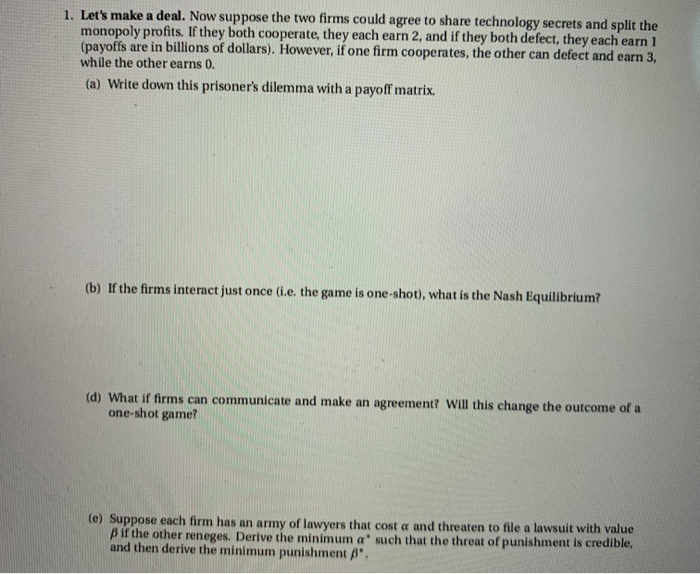1. Let's make a deal. Now suppose the two firms could agree to share technology secrets and split the monopoly profits. If they both cooperate, they each earn 2, and if they both defect, they each earn 1 (payoffs are in billions of dollars). However, if one firm cooperates, the other can defect and earn 3, while the other earns 0. (a) Write down this prisoner's dilemma with a payoff matrix. (b) If the firms interact just once (1.e. the...

• ### There are two firms in an industry. If both collude and set the monopolistic price, their...

There are two firms in an industry. If both collude and set the monopolistic price, their profits will be \$20 million each. If one firm sets the higher monopoly price and the other tries to get more business by charging a lower price, the high-priced firm earns \$6 million in profits and the low-priced firm earns \$30 million in profits. If both charge low prices, both earn \$10 million. What is the most likely outcome for the two firms? a....

• ### Suppose two firms cannot collude and compete in the Cournot Model. Market demand is Q =...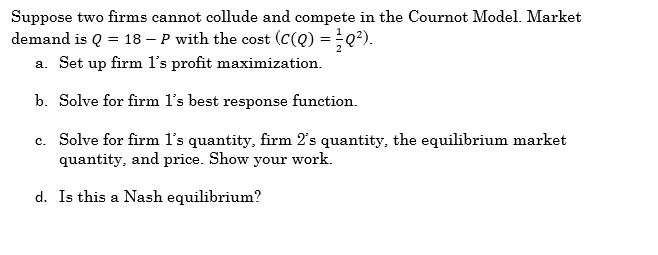Suppose two firms cannot collude and compete in the Cournot Model. Market demand is Q = 18 – P with the cost (c(Q) =*Q). a. Set up firm l's profit maximization. b. Solve for firm l's best response function. c. Solve for firm l's quantity, firm 2's quantity, the equilibrium market quantity, and price. Show your work. d. Is this a Nash equilibrium?

• ### The players are two firms in a duopoly, and a set of consumers. The two firms...

The players are two firms in a duopoly, and a set of consumers. The two firms produce a homogeneous good. The firms simultaneously choose their prices. Demand adjusts instantaneously according to the equation Q = 6 − p, Each firm has constant costs per unit of output. Firm 1’s cost per unit is 1, and firm 2’s cost per unit is 2. The firms’ payoffs are their profits. If the two firms’ prices are not equal, consumers will buy, according...